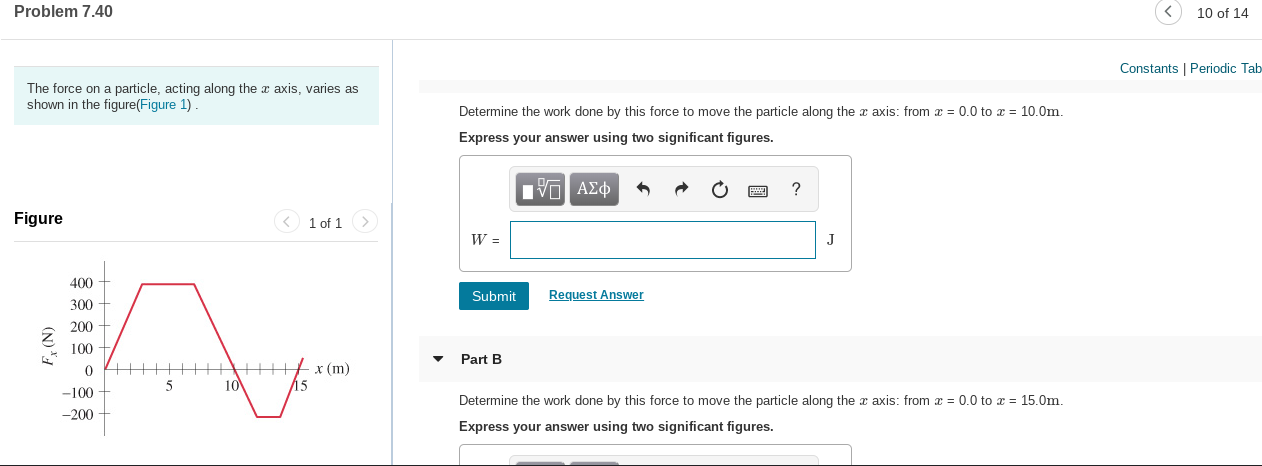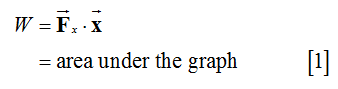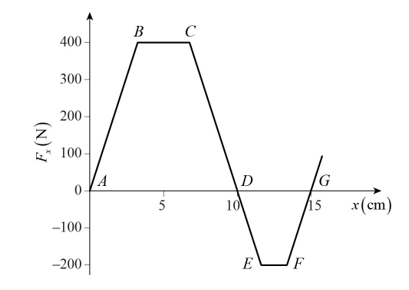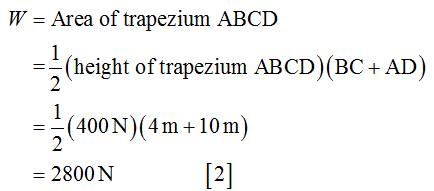# Problem 7.4010 of 14Constants | Periodic TabThe force on a particle, acting along the x axis, varies asshown in the figure(Figure 1).Determine the work done by this force to move the particle along the x axis: from x = 0.0 to x = 10.0m.Express your answer using two significant figures.V ΑΣφFigure< 1 of 1 >400SubmitRequest Answer300200100Part Bх (m)1510-100-200Determine the work done by this force to move the particle along the a axis: from x = 0.0 to x = 15.0m.Express your answer using two significant figures.(N) 4

Question
6 viewshelp_outlineImage TranscriptioncloseProblem 7.40 10 of 14 Constants | Periodic Tab The force on a particle, acting along the x axis, varies as shown in the figure(Figure 1). Determine the work done by this force to move the particle along the x axis: from x = 0.0 to x = 10.0m. Express your answer using two significant figures. V ΑΣφ Figure < 1 of 1 > 400 Submit Request Answer 300 200 100 Part B х (m) 15 10 -100 -200 Determine the work done by this force to move the particle along the a axis: from x = 0.0 to x = 15.0m. Express your answer using two significant figures. (N) 4 fullscreen
check_circle

Step 1

The work done by the force to move the particle along the x axis isStep 2

The work done by the force to move the particle along the x axis from x = 0.0 m to x = 10.0 m is...

### Want to see the full answer?

See Solution

#### Want to see this answer and more?

Solutions are written by subject experts who are available 24/7. Questions are typically answered within 1 hour.*

See Solution
*Response times may vary by subject and question.
Tagged in